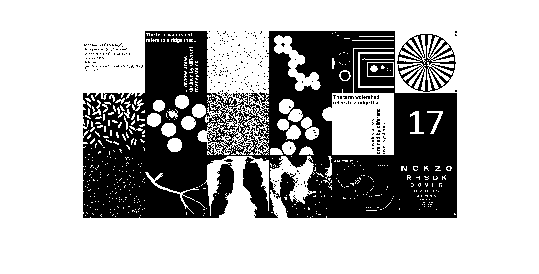# Isotropic dilation using the distance transform

Brett recently asked me about an image processing problem. One step in the problem involved finding out which pixels are within a certain distance of the foreground in a binary image. We experimented with a few methods, and I want to share the results with you. In particular, I want to show you a useful technique called isotropic dilation that can be achieved using the distance transform.

Let's start with a small 400-by-400 test image that has only a single foreground pixel in the center.

bw = false(400, 400);
bw(200, 200) = 1;
imshow(bw)Suppose we want to know which pixels are within 25 units of the foreground pixel? One common technique is to perform dilation with a disk.

se = strel('disk', 25);
bw2 = imdilate(bw, se);

This has a problem, though. If you read the documentation for strel carefully, you'll see that by default it computes a structuring element that is an approximation of a disk. You can see the effect of the approximation by looking at the output of the dilation:

imshow(bw2)You can avoid the approximation by passing in 0 as a third input argument to strel:

se2 = strel('disk', 25, 0);
bw3 = imdilate(bw, se2);
imshow(bw3)So why does strel use a disk approximation by default? Because it's usually a lot faster. Let's check it out on a larger test image (750-by-1500) with a lot of pixels in the foreground.

url = 'https://blogs.mathworks.com/images/steve/2010/binary_benchmark.png';
bw2 = imread(url);
imshow(bw2, 'InitialMagnification', 25)f = @() imdilate(bw2, se)
time_with_approximate_disk = timeit(f)
f =

@()imdilate(bw2,se)

time_with_approximate_disk =

0.0292


g = @() imdilate(bw2, se2)
time_with_exact_disk = timeit(g)
g =

@()imdilate(bw2,se2)

time_with_exact_disk =

1.4536



An alternative to using imdilate is to use bwdist to compute the distance transform and then threshold the result. The distance transform computes, for each pixel, the distance between that pixel and the nearest foreground pixel. Here's how it would work:

bw4 = bwdist(bw) <= 25;
imshow(bw4)The function bwdist uses a fast algorithm, so this works a lot faster than imdilate with an exact disk.

h = @() bwdist(bw2) <= 25;
time_using_distance_transform = timeit(h)
time_using_distance_transform =

0.0687



This technique of thresholding the distance transform is sometimes called isotropic dilation.

Have you made creative use of the distance transform in your work? Then post a comment here.

Published with MATLAB® 7.10

|

### Comments

To leave a comment, please click here to sign in to your MathWorks Account or create a new one.